# RD Sharma Solutions Class 9 Maths Chapter 24 Measures Of Central Tendency Exercise 24.3

Class 9 Chapter 24 – Measure of Central Tendency Exercise 24.3 solutions are provided here. RD Sharma Solutions for CBSE Class 9 are outlined keeping in mind the current CBSE syllabus, so that students can score good marks in the exams. In this section, students will learn about median of an ungrouped data. Click on the link below to get your pdf for free.

## Download PDF of RD Sharma Solutions for Class 9 Maths Chapter 24 Measure of Central Tendency Exercise 24.3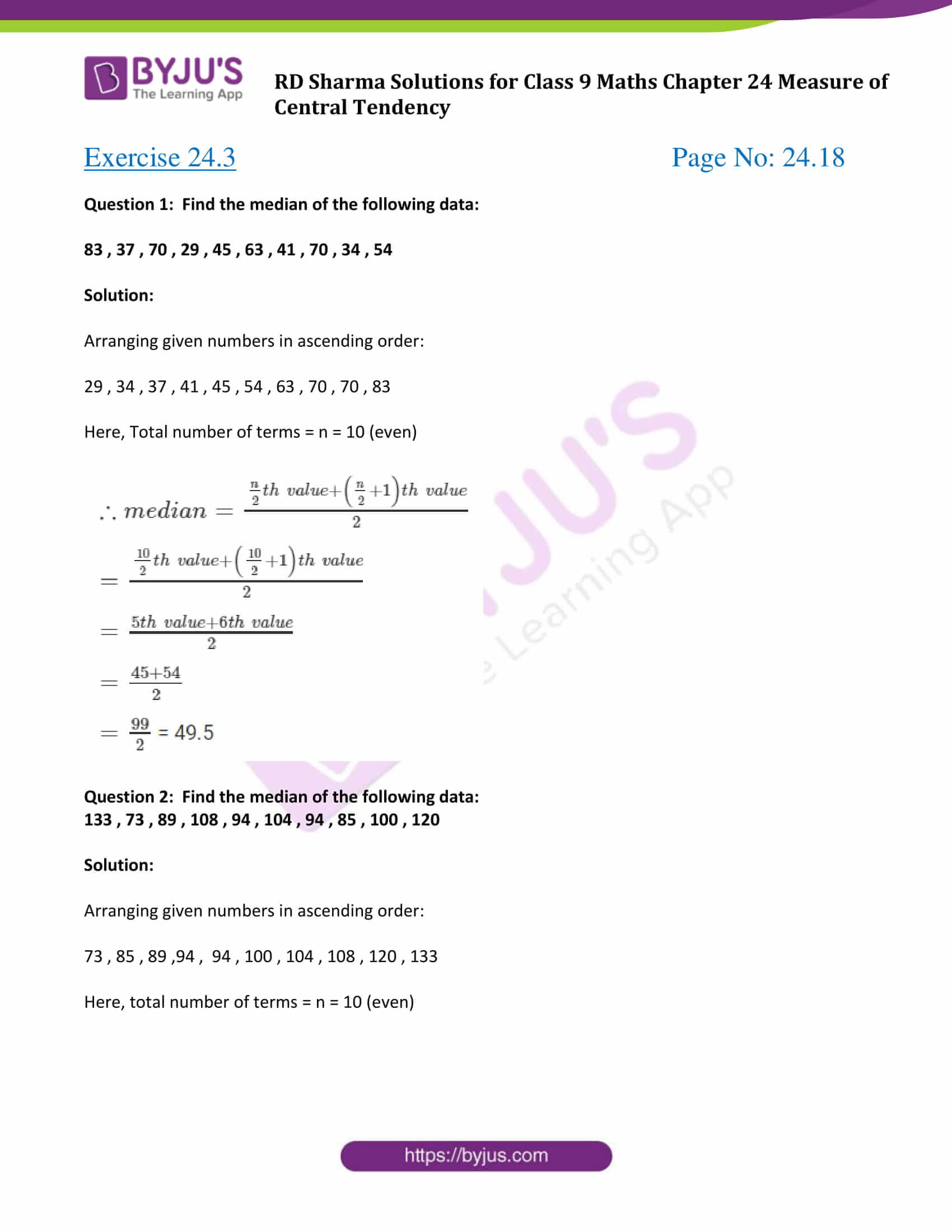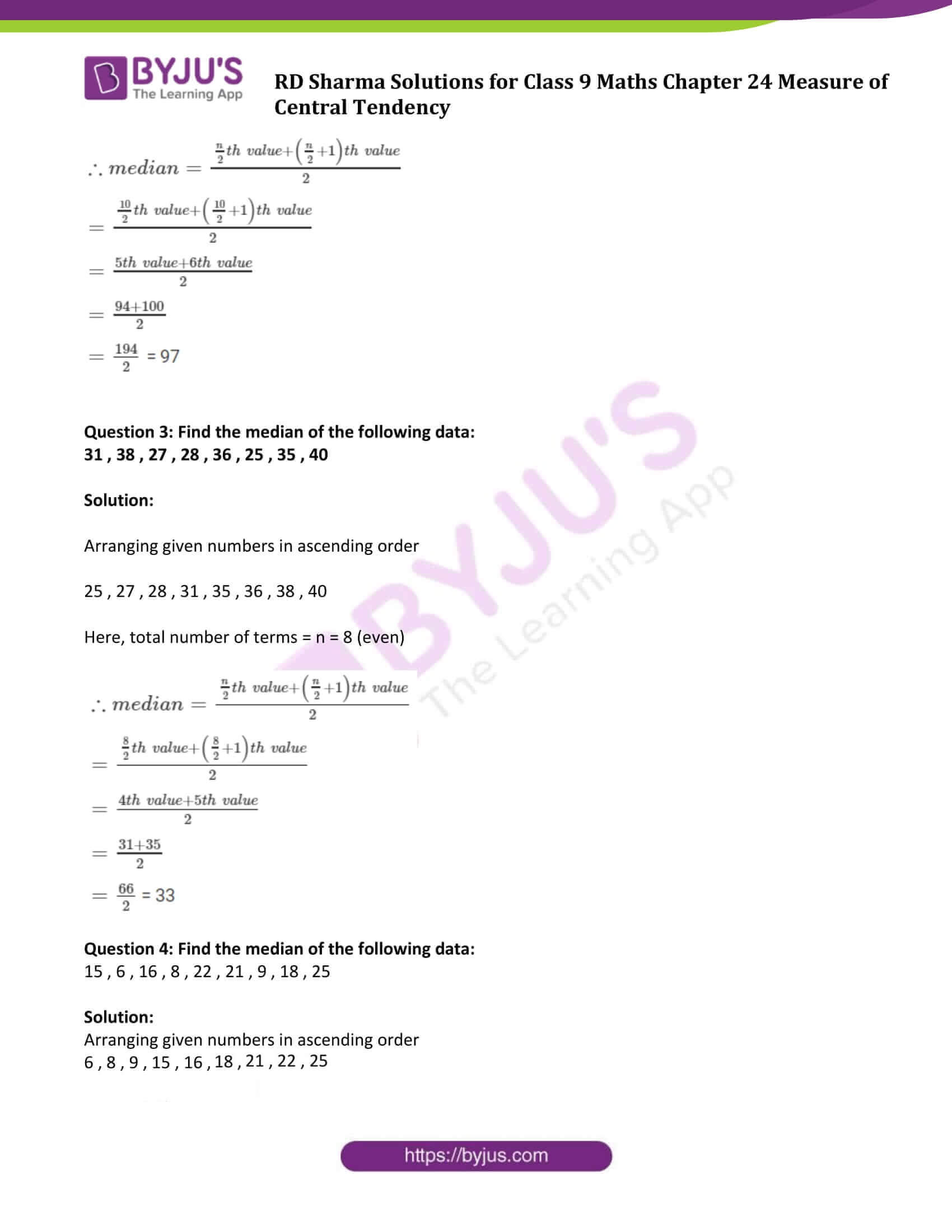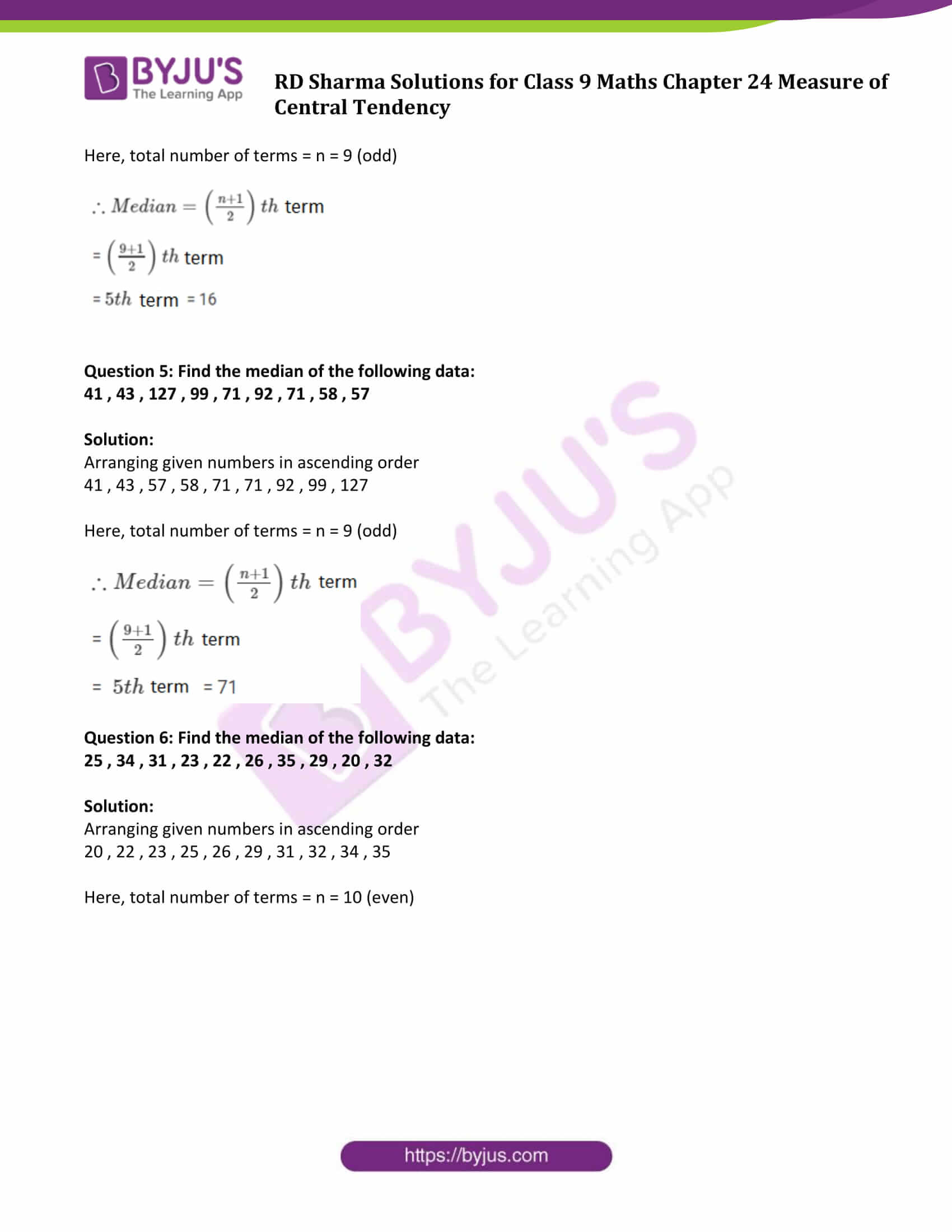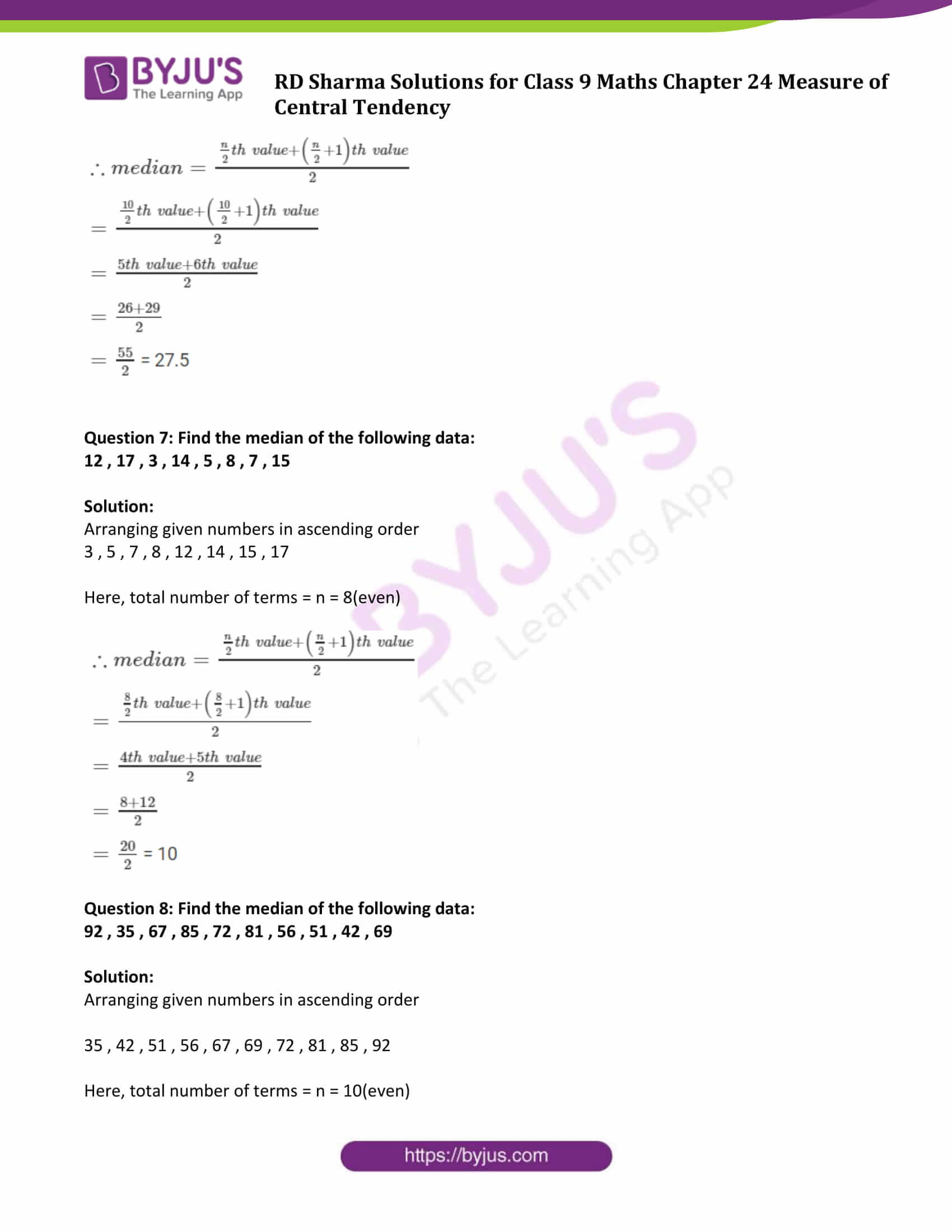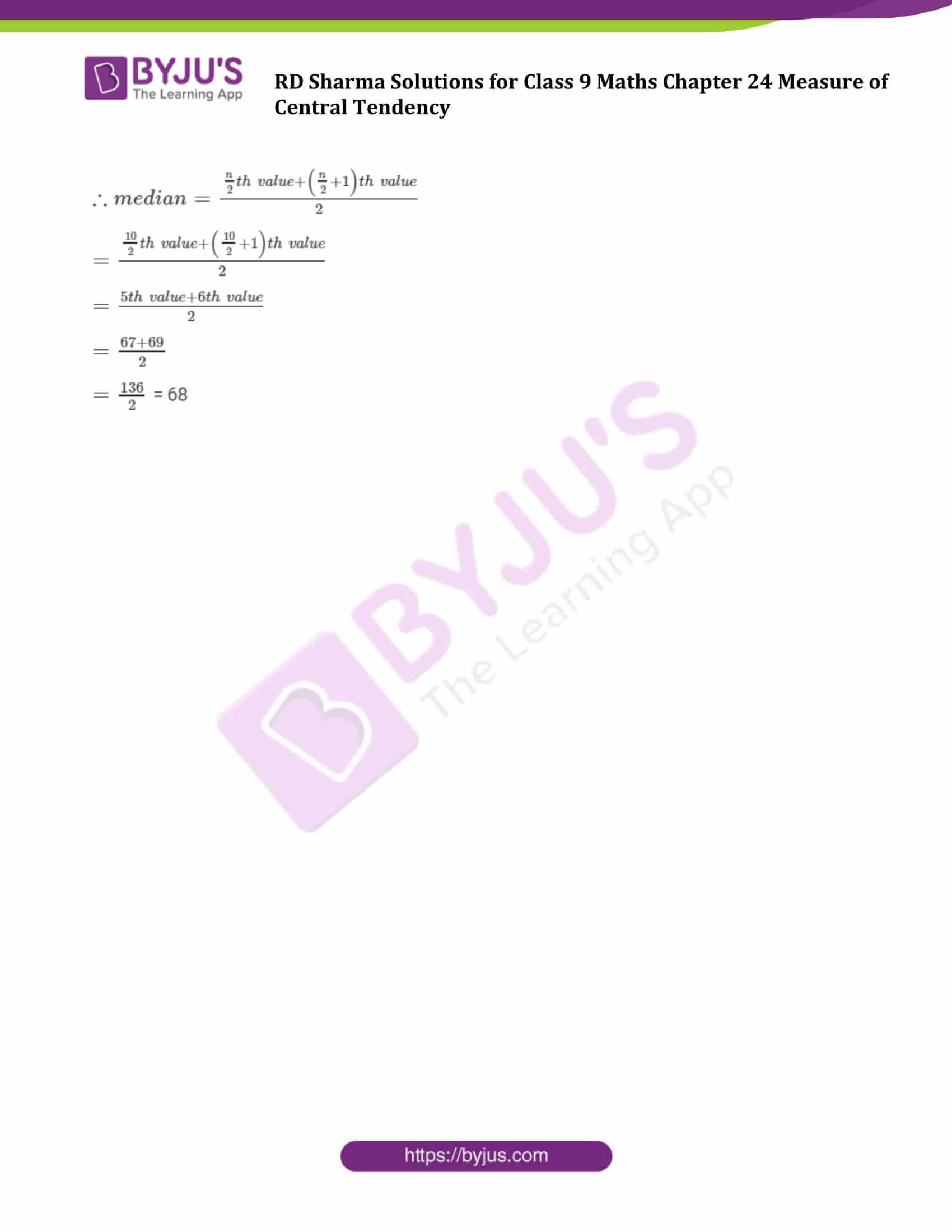### Access Answers to Maths RD Sharma Solutions for Class 9 Chapter 24 Measure of Central Tendency Exercise 24.3 Page number 24.18

Question 1: Find the median of the following data:

83 , 37 , 70 , 29 , 45 , 63 , 41 , 70 , 34 , 54

Solution:

Arranging given numbers in ascending order:

29 , 34 , 37 , 41 , 45 , 54 , 63 , 70 , 70 , 83

Here, Total number of terms = n = 10 (even)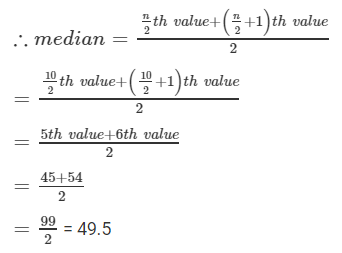Question 2: Find the median of the following data:

133 , 73 , 89 , 108 , 94 , 104 , 94 , 85 , 100 , 120

Solution:

Arranging given numbers in ascending order:

73 , 85 , 89 ,94 , 94 , 100 , 104 , 108 , 120 , 133

Here, total number of terms = n = 10 (even)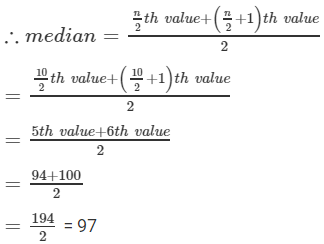Question 3: Find the median of the following data:

31 , 38 , 27 , 28 , 36 , 25 , 35 , 40

Solution:

Arranging given numbers in ascending order

25 , 27 , 28 , 31 , 35 , 36 , 38 , 40

Here, total number of terms = n = 8 (even)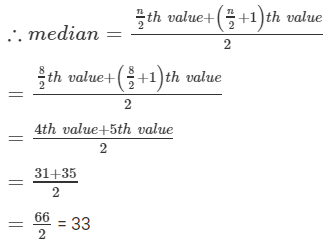Question 4: Find the median of the following data:

15 , 6 , 16 , 8 , 22 , 21 , 9 , 18 , 25

Solution:

Arranging given numbers in ascending order

6 , 8 , 9 , 15 , 16 , 18, 21 , 22 , 25

Here, total number of terms = n = 9 (odd)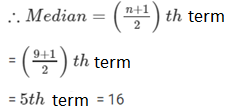Question 5: Find the median of the following data:

41 , 43 , 127 , 99 , 71 , 92 , 71 , 58 , 57

Solution:

Arranging given numbers in ascending order

41 , 43 , 57 , 58 , 71 , 71 , 92 , 99 , 127

Here, total number of terms = n = 9 (odd)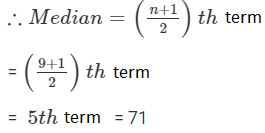Question 6: Find the median of the following data:

25 , 34 , 31 , 23 , 22 , 26 , 35 , 29 , 20 , 32

Solution:

Arranging given numbers in ascending order

20 , 22 , 23 , 25 , 26 , 29 , 31 , 32 , 34 , 35

Here, total number of terms = n = 10 (even)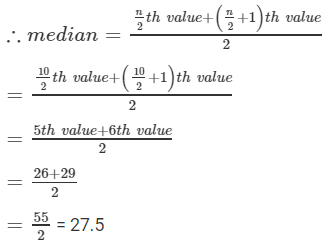Question 7: Find the median of the following data:

12 , 17 , 3 , 14 , 5 , 8 , 7 , 15

Solution:

Arranging given numbers in ascending order

3 , 5 , 7 , 8 , 12 , 14 , 15 , 17

Here, total number of terms = n = 8(even)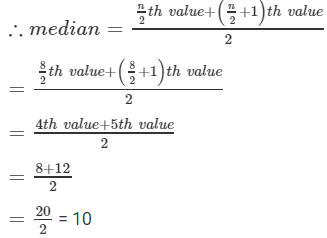Question 8: Find the median of the following data:

92 , 35 , 67 , 85 , 72 , 81 , 56 , 51 , 42 , 69

Solution:

Arranging given numbers in ascending order

35 , 42 , 51 , 56 , 67 , 69 , 72 , 81 , 85 , 92

Here, total number of terms = n = 10(even)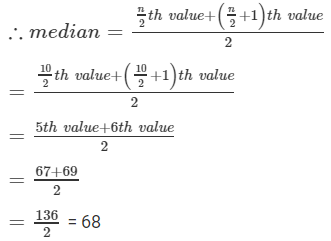## RD Sharma Solutions for Class 9 Maths Chapter 24 Measures of Central Tendency Exercise 24.3

RD Sharma solutions Class 9 Maths Chapter 24 Measures of Central Tendency Exercise 24.3 is based on following topics:

• Median
• Median of an ungrouped data